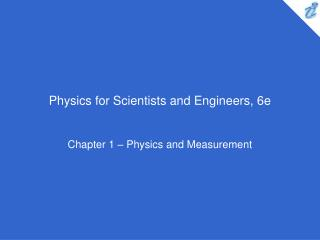DownloadDownload PresentationPhysics for Scientists and Engineers, 6e

# Physics for Scientists and Engineers, 6e

Télécharger la présentation## Physics for Scientists and Engineers, 6e

- - - - - - - - - - - - - - - - - - - - - - - - - - - E N D - - - - - - - - - - - - - - - - - - - - - - - - - - -
##### Presentation Transcript

1. Physics for Scientists and Engineers, 6e Chapter 1 – Physics and Measurement

2. In a machine shop, two cams are produced, one of aluminum, one of iron. Both cams have the same mass. Which cam is larger? • the aluminum cam • the iron cam • Both cams have the same size.

3. Because the density of aluminum is smaller than that of iron, a larger volume of aluminum is required for a given mass than iron.

4. True or False: Dimensional analysis can give you the numerical value of constants of proportionality that may appear in an algebraic expression. • True • False

5. False. Dimensional analysis gives the units of the proportionality constant but provides no information about its numerical value. To determine its numerical value requires either experimental data or geometrical reasoning. For example, in the generation of the equation x = ½ at2, because the factor ½ is dimensionless, there is no way of determining it using dimensional analysis.

6. The distance between two cities is 100 mi. The number of kilometers between the two cities is • smaller than 100 • larger than 100 • equal to 100

7. Because kilometers are shorter than miles, a larger number of kilometers is required for a given distance than miles.